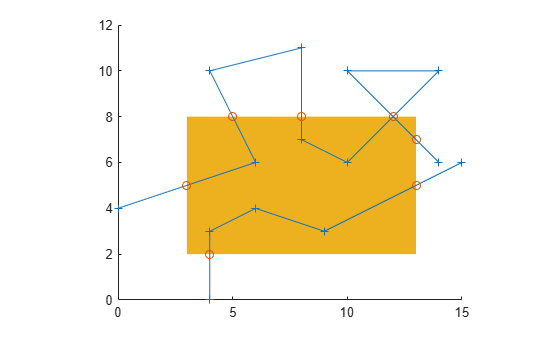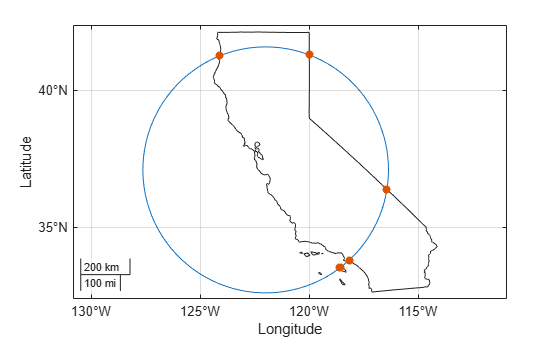polyxpoly

Intersection points for lines or polygon edges

Description

example

[xi,yi] = polyxpoly(x1,y1,x2,y2) returns the intersection points of two polylines in a planar, Cartesian system, with vertices defined by x1, y1, x2 and y2. The output arguments, xi and yi, contain the x- and y-coordinates of each point at which a segment of the first polyline intersects a segment of the second. In the case of overlapping, collinear segments, the intersection is actually a line segment rather than a point, and both endpoints are included in xi, yi.

[xi,yi,ii] = polyxpoly(___) returns a two-column array of line segment indices corresponding to the intersection points. The k-th row of ii indicates which polyline segments give rise to the intersection point xi(k), yi(k).

To remember how these indices work, just think of segments and vertices as fence sections and posts. The i-th fence section connects the i-th post to the (i+1)-th post. In general, letting i and j denote the scalar values comprised by the k-th row of ii, the intersection indicated by that row occurs where the i-th segment of the first polyline intersects the j-th segment of the second polyline. But when an intersection falls precisely on a vertex of the first polyline, then i is the index of that vertex. Likewise with the second polyline and the index j. In the case of an intersection at the i-th vertex of the first line, for example, xi(k) equals x1(i) and yi(k) equals y1(i). In the case of intersections between vertices, i and j can be interpreted as follows: the segment connecting x1(i), y1(i) to x1(i+1), y1(i+1) intersects the segment connecting x2(j), y2(j) to x2(j+1), y2(j+1) at the point xi(k), yi(k).

example

[xi,yi] = polyxpoly(___,'unique') filters out duplicate intersections, which may result if the input polylines are self-intersecting.

Examples

collapse all

Define and fill a rectangular area in the plane.

xlimit = [3 13];
ylimit = [2  8];
xbox = xlimit([1 1 2 2 1]);
ybox = ylimit([1 2 2 1 1]);
mapshow(xbox,ybox,'DisplayType','polygon','LineStyle','none')

Define and display a two-part polyline.

x = [0 6  4  8 8 10 14 10 14 NaN 4 4 6 9 15];
y = [4 6 10 11 7  6 10 10  6 NaN 0 3 4 3  6];
mapshow(x,y,'Marker','+')

Intersect the polyline with the rectangle.

[xi,yi] = polyxpoly(x,y,xbox,ybox);
mapshow(xi,yi,'DisplayType','point','Marker','o')Display the intersection points; note that the point (12, 8) appears twice because of a self-intersection near the end of the first part of the polyline.

[xi yi]
ans = 8×2

3.0000    5.0000
5.0000    8.0000
8.0000    8.0000
12.0000    8.0000
12.0000    8.0000
13.0000    7.0000
13.0000    5.0000
4.0000    2.0000

You can suppress this duplicate point by using the 'unique' option.

[xi,yi] = polyxpoly(x,y,xbox,ybox,'unique');
[xi yi]
ans = 7×2

3.0000    5.0000
5.0000    8.0000
8.0000    8.0000
12.0000    8.0000
13.0000    7.0000
13.0000    5.0000
4.0000    2.0000

Read state polygons into a geospatial table. Create a subtable that contains the California polygon. Display the polygon on a map.

row = states.Name == "California";
california = states(row,:);

figure
usamap("california")
geoshow(california,"FaceColor","none")

Define a small circle centered off the coast of California.

lat0 = 37;
lon0 = -122;
plotm(lat0,lon0,"r*")
plotm(latc,lonc,"r")

Extract the latitude and longitude coordinates of the California polygon from the geospatial table.

T = geotable2table(california,["Latitude","Longitude"]);
[lat,lon] = polyjoin(T.Latitude',T.Longitude');

Find the intersection points between the state of California and the small circle.

[loni,lati] = polyxpoly(lon,lat,lonc,latc);
plotm(lati,loni,"bo")Input Arguments

collapse all

x- or y-coordinates of points in the first or second polyline, specified as a numeric vector. For a given polyline, the x- and y-coordinate vectors must be the same length.

Output Arguments

collapse all

x- or y-coordinates of intersection points, specified as a numeric column vector.

Line segment indices of intersection points, specified as a numeric vector.

Tips

• If the spacing between points is large, the intersections calculated by the polyxpoly function and the intersections shown on a map display might be different. This is a result of differences between straight lines in the unprojected and projected coordinates. Similarly, there might be differences between the polyxpoly result and intersections that assume great circles or rhumb lines between points.